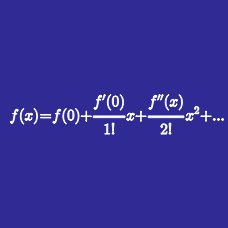Calculus

# Taylor Series Manipulation

Determine the Taylor series for the function

$f(x)=\sinh x ~\text{ centered at }~ x=0.$

Determine the Taylor series for the function

$f(x)=\cosh x ~\text{ centered at }~ x=0.$

Determine the Taylor series for the function

$f(x)=e^{x^2} ~\text{ centered at }~ x=0.$

Determine the Taylor series for the function

$f(x)=\frac{1}{(1 - x)^2} ~\text{ centered at }~ x=0 ~\text{ for }~ \left |x \right |<1.$

Determine the Taylor series for the function

$f(x)=\sin{x^2} ~\text{ centered at }~ x=0.$

×